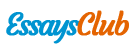# Correlation: Point Biserial Model

Autor:   •  June 12, 2019  •  Essay  •  415 Words (2 Pages)  •  1,179 Views

Page 1 of 2

 -- Saturday, February 02, 2019 -- 14:46:34

t tests - Correlation: Point biserial model

Analysis: A priori: Compute required sample size

Input: Tail(s) = One

Effect size |ρ| = 0.5

α err prob = 0.05

Power (1-β err prob) = 0.8

Output: Noncentrality parameter δ = 2.6457513

Critical t = 1.7291328

Df = 19

Total sample size = 21

Actual power = 0.8172279

 -- Saturday, February 02, 2019 -- 14:46:44

t tests - Means: Difference between two independent means (two groups)

Analysis: A priori: Compute required sample size

Input: Tail(s) = One

Effect size d = 0.5

α err prob = 0.05

Power (1-β err prob) = 0.95

Allocation ratio N2/N1 = 1

Output: Noncentrality parameter δ = 3.3166248

Critical t = 1.6536580

Df = 174

Sample size group 1 = 88

Sample size group 2 = 88

Total sample size = 176

Actual power = 0.9514254

t tests - Means: Difference between two independent means (two groups)

Analysis: A priori: Compute required sample size

Input: Tail(s) = Two

Effect size d = 0.5

α err prob = 0.05

Power (1-β err prob) = 0.80

Allocation ratio N2/N1 = 1

Output: Noncentrality parameter δ = 2.8284271

Critical t = 1.9789706

Df = 126

Sample size group 1 = 64

Sample size group 2 = 64

Total sample size = 128

Actual power = 0.8014596

 -- Saturday, February 02, 2019 -- 15:58:47

t tests - Means: Difference between two independent means (two groups)

Analysis: A priori: Compute required sample size

Input: Tail(s) = Two

Effect size d = 0.5

α err prob = 0.05

Power (1-β err prob) = 0.8

Allocation ratio N2/N1 = 1

Output: Noncentrality parameter δ = 2.8284271

Critical t = 1.9789706

Df = 126

Sample size group 1 = 64

Sample size group 2 = 64

Total sample size = 128

Actual power = 0.8014596

 -- Saturday, February 02, 2019 -- 15:58:50

t tests - Means: Difference between two independent means (two groups)

Analysis: A priori: Compute required sample size

Input: Tail(s) = Two

Effect size d = 0.5

α err prob = 0.05

Power (1-β err prob) = 0.80

Allocation ratio N2/N1 = 1

Output: Noncentrality parameter δ = 2.8284271

Critical t = 1.9789706

Df = 126

Sample size group 1 = 64

Sample size group 2 = 64

Total sample size = 128

Actual power = 0.8014596

 -- Saturday, February 02, 2019 -- 15:58:53

t tests -

...